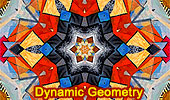Dynamic Geometry, Interactive Geometry Software IGS, Geometric ConstructionsDynamic Geometry  or  Interactive Geometry Software are computer programs which allow one to create and then manipulate geometric constructions. GeoGebra Constructions Index HTML5 Animation for Tablets (iPad, Nexus..) Dynamic Geometry 1470. Tangential Quadrilateral, Incircles, Tangent, Parallel, Rhombus, Step-by-step Illustration. Dynamic Geometry 1469. Triangle, Circumradius, Inradius, Midpoints, Arcs, Sum of Distances, Step-by-step Illustration. Dynamic Geometry 1468. Steiner's Theorem, Triangle, Circumradius, Inradius, Sum of Exradii, Step-by-step Illustration. Geometry Problem 1466. Tangential Quadrilateral, Newton Line, Incenter, Midpoint, Diagonal, Collinear Points. Step-by-step Illustration using GeoGebra. Geometry Problem 1465. Tangential Quadrilateral, Incenter, Inscribed Circle, Equal Sum of Areas. Step-by-step Illustration using GeoGebra. Geometry Problem 1464. Quadrilateral, Interior Point, Midpoint of Sides, Equal Sum of Areas. Step-by-step Illustration using GeoGebra. Geometry Problem 1463. Parallelogram, Interior Point, Opposite Triangles with Equal Sum of Areas. Step-by-step Illustration using GeoGebra. Geometry Problem 1462. Newton-Line, Newton-Gauss Line, Complete Quadrilateral, Midpoints of Sides and Diagonals, Collinear Points. Dynamic Geometry 1460. Newton-Gauss Line, Complete Quadrilateral, Midpoints of Diagonals, Collinear Points, Step-by-step Illustration using GeoGebra. Geometry Problem 1459. Two Triangles, Orthocenter, Midpoint, Perpendicular, Step-by-step Illustration using GeoGebra. Geometry Problem 1458. Triangle, Incircles, Excircle, Area, Step-by-step Illustration using GeoGebra. Geometry Problem 1457. Altitudes, Circles, Similarity, Product of the Inradii Lengths, iPad. Step-by-step illustration using GeoGebra. Geometry Problem 1456. Altitudes, Orthic Triangle, Circumcircle, Parallel lines, Similarity, Area, iPad. Step-by-step illustration using GeoGebra. Geometry Problem 1455. Nagel Point, Excircles, Incircle, Congruent Segments, iPad. Step-by-step illustration using GeoGebra. Geometry Problem 1454. Intersecting Circles, Perpendicular Lines, Cyclic Quadrilateral, Collinear Points, iPad. Step-by-step illustration using GeoGebra. Dynamic Geometry 1452. Japanese Theorem, Sangaku, Cyclic Quadrilateral, Incenter, Rectangle, Inradius. Step-by-step illustration using GeoGebra. Dynamic Geometry 1451. Orthopole of a Line. Step-by-step illustration using GeoGebra. Dynamic Geometry 1450. Ortholine, Steiner Line, Complete Quadrilateral, Collineal Orthocenters. Step-by-step illustration using GeoGebra. Dynamic Geometry 1449. Salmon Line. Step-by-step illustration using GeoGebra. Dynamic Geometry 1448. Simson Line. Step-by-step illustration using GeoGebra. Dynamic Geometry Problem 1447. Outer Vecten Point. Step-by-step illustration using GeoGebra. Dynamic Geometry Problem 1446. Lemoine Line, triangle, circumcircle, tangent, collinear points. Step-by-step animation using GeoGebra. Dynamic Geometry Problem 1445. Van Aubel's theorem, Quadrilateral and Four Squares, Centers. Step-by-step animation using GeoGebra. iPad Apps: Apollonius, Interactive Geometry Software IGS Index. Theorems and Problems with Apollonius for iPad. Geometry Designer for iPad, iPhone, iPod - Index Dynamic Geometry. Interactive geometry software for the iPhone and iPod Touch. Video: the first construction is a regular hexagon given its center and one vertex. The second construction is the nine-point circle. Dynamic Geometry: Exeter PointTriangle, Median, Circumcircle, Concurrent Lines, Euler Line, GeoGebra, HTML5 Animation for Tablets. Wolfram Demonstrations Project & GoGeometry. Interact with demonstrations using the free Mathematica Player GeoGebra, Dynamic Geometry, Euclidean Egg with 8 Arcs in 5 Steps, Step-by-Step Construction HTML5 Animation for Tablets (iPad, Nexus) GeoGebra, Dynamic Geometry: Internal Feuerbach Point HTML5 Animation for Tablets (iPad, Nexus..) GeoGebra, Dynamic Geometry, Euclidean Egg, Step-by-Step Construction HTML5 Animation for Tablets (iPad, Nexus) GeoGebra, Dynamic Geometry: Nine Point Circle HTML5 Animation for Tablets (iPad, Nexus..) GeoGebra, Dynamic Geometry: Lemoine Line of a Triangle. HTML5 Animation for Tablets (iPad, Nexus..) GeoGebra, Dynamic Geometry: Simson Line of a Triangle. HTML5 Animation for Tablets (iPad, Nexus..) GeoGebra, Dynamic Geometry: Euler Line of a Triangle. HTML5 Animation for Tablets (iPad, Nexus..) GeoGebra, Dynamic Geometry: Incenter and Incircle of a Triangle. HTML5 Animation for Tablets (iPad, Nexus..) GeoGebra, Dynamic Geometry: Centroid of a Triangle. HTML5 Animation for Tablets (iPad, Nexus..) GeoGebra, Dynamic Geometry: Circumcenter and Circumcircle of a Triangle. HTML5 Animation for Tablets (iPad, Nexus..) Archimedes Arbelos and Square 2. Dynamic Geometry Software. Step-by-Step construction, Manipulation, and animation. Go to page: Previous | 1 | 2 | 3 | 4 | 5 | Next

Home | Sitemap | Go Geometry Education | SearchGeometry | Post a comment | Email | by Antonio Gutierrez
Last updated: Apr 16, 2020